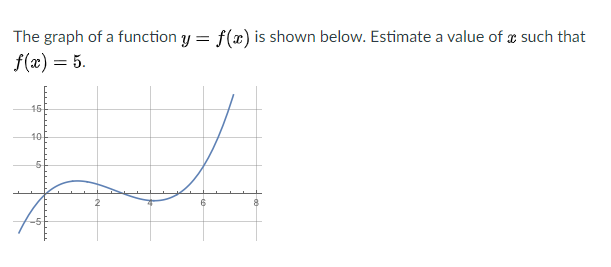# The graph of a function y = f(x) is shown below. Estimate a value of æ such that f(x) = 5. 16 10

Question

The graph of a function y = f ( x ) is shown below. Estimate a value of x such that f ( x ) = 5.help_outlineImage TranscriptioncloseThe graph of a function y = f(x) is shown below. Estimate a value of æ such that f(x) = 5. 16 10 fullscreen

### Want to see this answer and more?

Experts are waiting 24/7 to provide step-by-step solutions in as fast as 30 minutes!*

*Response times may vary by subject and question complexity. Median response time is 34 minutes for paid subscribers and may be longer for promotional offers.
Tagged in
Math
Calculus

### Functions